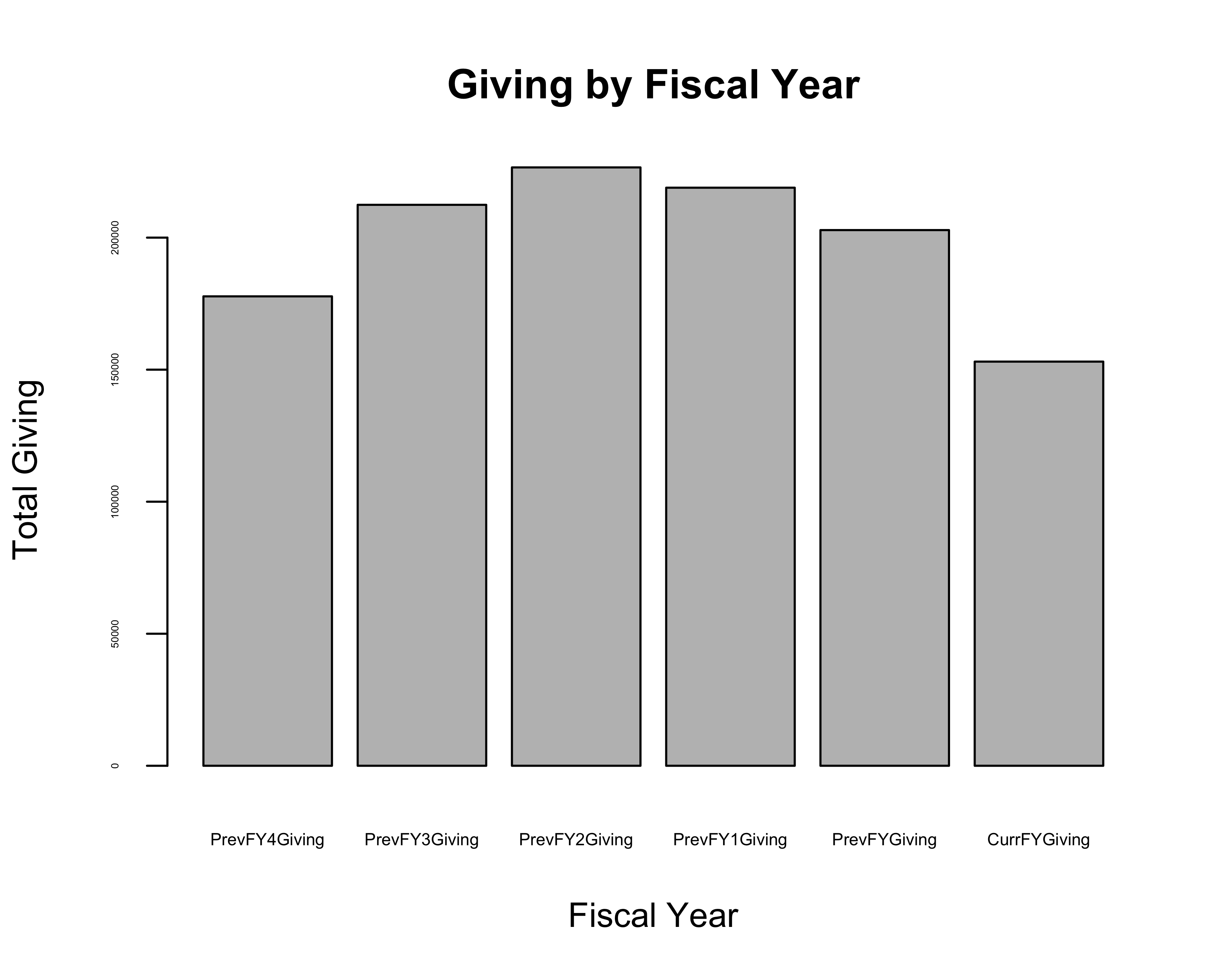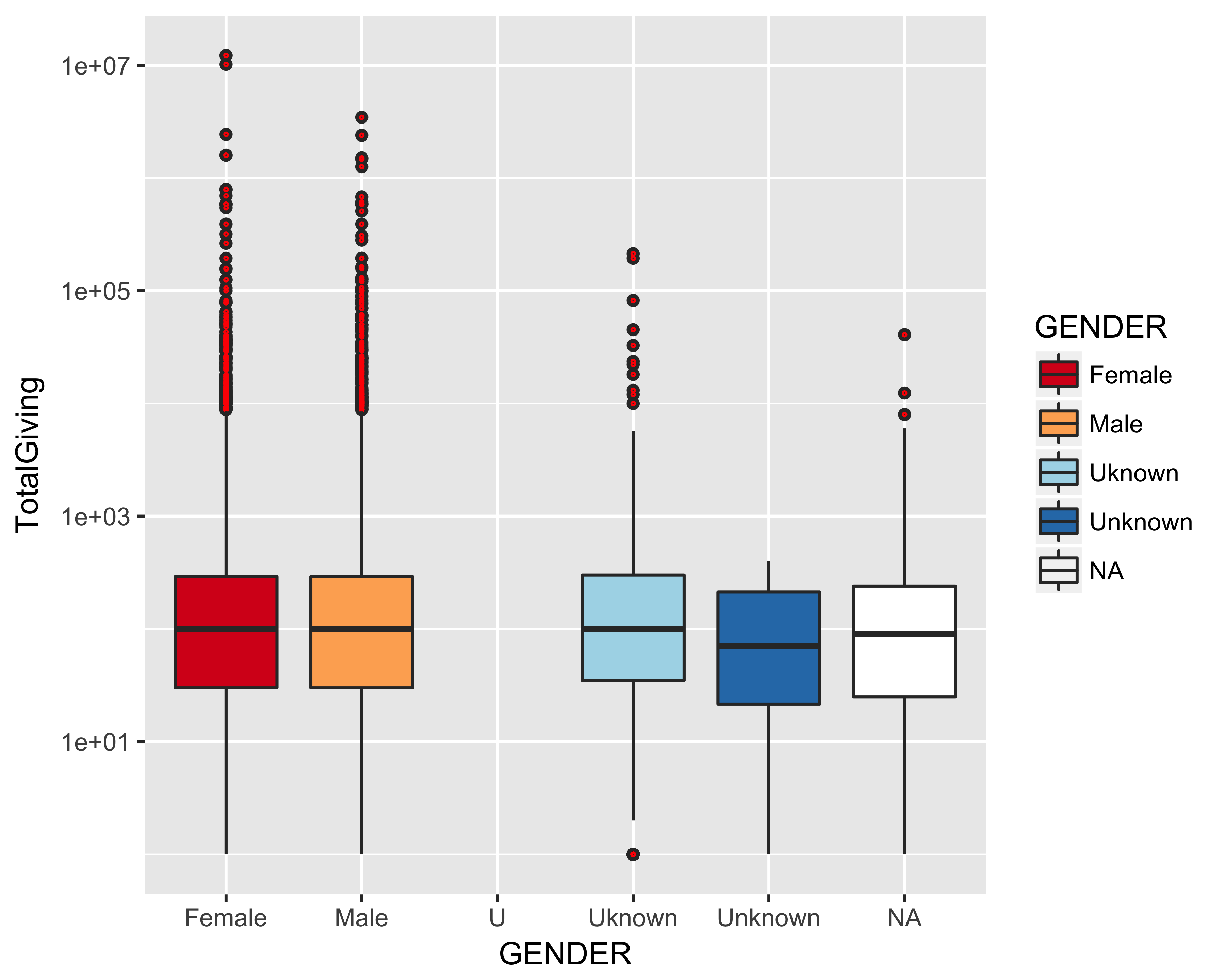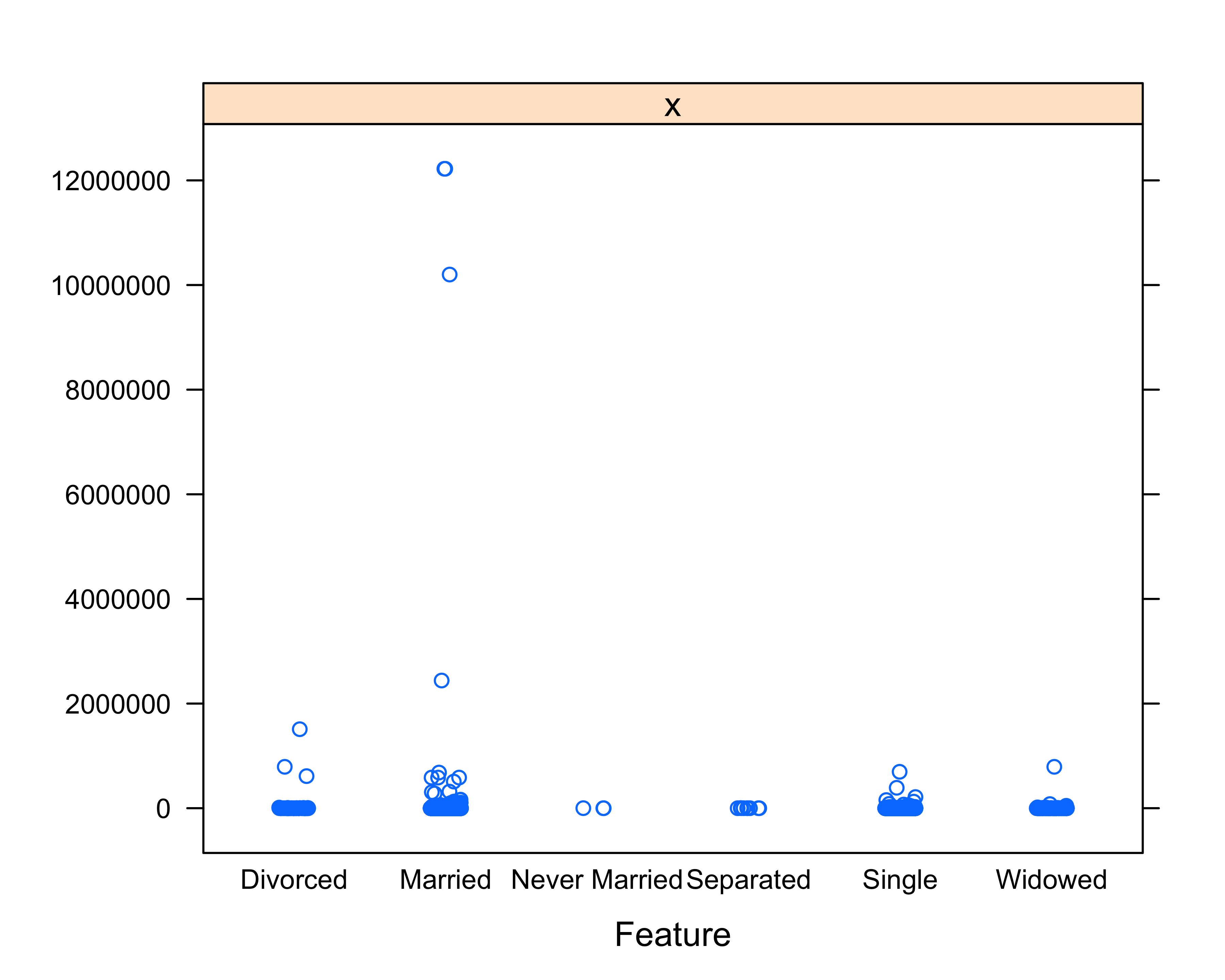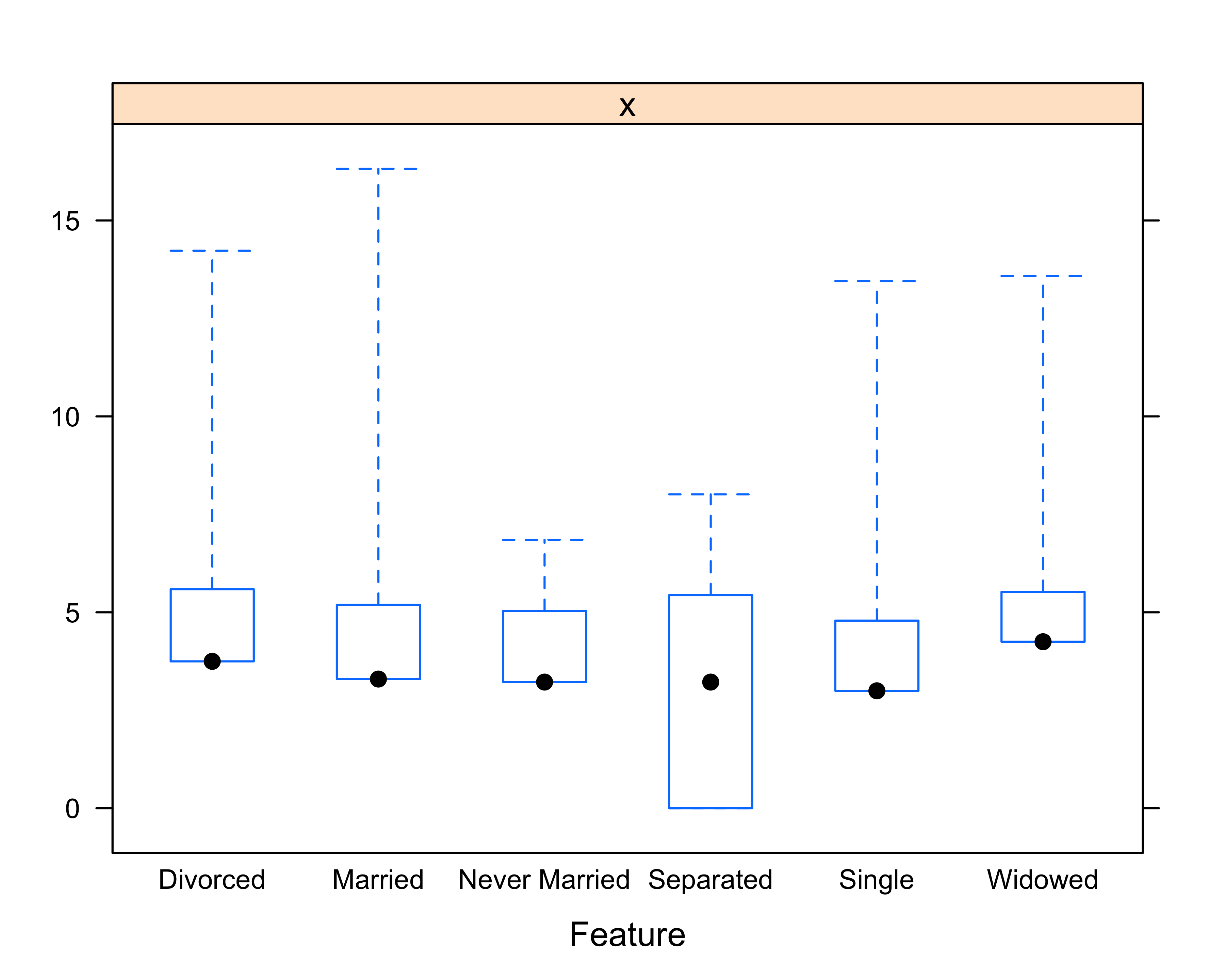# Chapter 9 Exploratory Data Analysis

Exploratory data analysis (also known as EDA) describes a broad set of statistical and graphical techniques that summarize information to guide further analysis and data collection processes. Exploratory data analysis lays the foundation for more formal analyses such as model building and statistical hypothesis testing. Since exploratory data analysis is somewhat loosely defined, it may also be useful to think of it as an intermediate or staging process that involves a variety of data analysis and visualization techniques that you can use to evaluate, guide, and refine your analyses, data collection, and model building.

John W. Tukey, a prominent American mathematician best known for creating the box plot data visualization (and perhaps less well known for coining the term “bit” while developing computational statistical methods at Bell Labs), is the author of Exploratory Data Analysis (Tukey 1977). Tukey cautioned against jumping headfirst into statistical hypothesis testing without first exploring and analyzing data beforehand.

Tukey’s contributions to statistical computing and EDA are especially significant because they inspired the development of the `S` programming language at Bell Labs, which is the precursor of the `R` programming language used in this book. `S`, which was launched in 1976 and evolved into `S-PLUS` (1988) and eventually `R` (1993), accelerated the course of statistical computing by introducing revolutionary graphical capabilities to identify outliers, trends, and insights.

EDA includes many graphical techniques that will be covered extensively in the Data Visualization chapter. In this chapter, we will provide a few graphical methods to introduce you to statistical thinking and inspire you to think about how you can use `R` to identify outliers, trends, and meaningful patterns in your data.

Let’s take a dive into our sample donor file and introduce you to some exploratory data analysis concepts.

``````# Load readr and dplyr libraries
library(dplyr)

You can use the `head` or `tail` function to select the first or last `n` rows of data, where `n` specifies the number of rows.

``````# Show first 10 rows

# Show last 10 rows
tail(donor_data, n = 10)``````

## 9.2 Glimpse

Since we loaded the `dplyr` library, let’s use the `glimpse` function to take a look at the features in our sample donor file:

``````# Show loaded data
glimpse(donor_data)``````

## 9.3 Slice and Dice

The following are two additional ways to select and display the first 10 rows of data using either manual row selection or the `slice` function from `dplyr`.

``````# Select first 10 rows
donor_data[1:10, ]

# Select first 10 rows using dplyr
slice(donor_data, 1:10)``````

## 9.4 Indexing Notation

In addition to selecting rows, you can select specific columns in data frame indexing [rows, columns] notation.

``````# Select first 10 rows of the first 3
# columns: [rows, columns]
donor_data[1:10, 1:3]

# Select first 3 columns of the first 10 rows using
# dplyr
select(donor_data, 1:3) %>% slice(1:10)``````

## 9.5 Deselect Rows and Columns

You can also use the “-” character to indicate all rows or columns except those meeting certain criteria. The following command selects the first three rows and excludes columns 1–6 and 9–23, which leaves only columns 7 and 8 (Alumnus and Parent Indicators).

``````# Select first 3 rows of all columns except columns
# 1-6 and columns 9-23
donor_data[1:3, -c(1:6, 9:23)]
#> # A tibble: 3 x 2
#>   ALUMNUS_IND PARENT_IND
#>         <chr>      <chr>
#> 1           N          N
#> 2           Y          N
#> 3           N          N``````

## 9.6 Select Columns by Name

In addition to column (or feature) selection by column number, you can also specify the column name using the `\$` operator.

``````# Select by column name, calculate sum and store
# into TotalGivingSum
TotalGivingSum <- sum(donor_data\$TotalGiving)

# Print TotalGivingSum
TotalGivingSum
#>  81560838``````

## 9.7 Summary

Another useful way to explore your data is to invoke the `summary` function, which produces summary statistics for each variable within your data object. The output displayed will depend on the type of data object. This function is especially useful when working with numeric data because the output displays the following summary statistics for each variable: minimum, maximum, first quartile (25th percentile), median (50th percentile), mean, and third quartile (75th percentile) values. Give `summary` a try within the `R` console using the following command.

``````# Summarize "data" by individual variable
summary(donor_data)``````

Let’s use some statistical measures of central tendency to identify average gift size.

``````# Print average, median, standard deviation and
# quantiles of giving
mean(donor_data\$TotalGiving)
#>  2364
median(donor_data\$TotalGiving)
#>  25``````

It’s the tale of two averages. Using the `mean` function, we can see that the average gift size (mean) in our donor file is \$2,364, and the median is \$25. Let’s further explore and compare both statistical measures of average gift size in this example.

While both calculations report the “average” gift size, the mean value of \$2,364 is significantly larger than the median value of \$25, which indicates there are some large gifts (outliers) strongly influencing the overall average (mean) gift size. The median value (\$25), by contrast, is calculated by arranging all of the gift size values in order from smallest to largest and selecting the middle (or median) value.

In cases where there are a few extremely low or high values (outliers) in your data, the median value is useful because it is robust against outlier values and may offer a better way to represent what is a typical value in your data set. In this example, the median value of \$25 likely provides a better sense of the average gift size.

Let’s use some additional statistical methods to explore the distribution (spread) of this data.

``````# Print average, median, standard deviation and
# quantiles of giving
sd(donor_data\$TotalGiving)
#>  113801
quantile(donor_data\$TotalGiving)
#>       0%      25%      50%      75%     100%
#>        0        0       25      144 12221854``````

Using the `quantile` function, we learn that 75% of the donors in our synthetic data set are giving \$144 or below.

You can also use the `quantile` function to determine the 90th, 95th, and 99th percentiles of giving levels in the donor file.

``````# Print average, median, standard deviation and
# quantiles of giving
quantile(donor_data\$TotalGiving,
probs = c(0.9, 0.95, 0.99))
#>  90%  95%  99%
#>  525 1309 9599``````

Using the `quantile` function with an additional array of probability parameters, we determine that 90% of donors gave \$525 or below, 95% of donors gave \$1,309 or below, and that 99% of donors gave below \$10,000 in this data set.

## 9.10 Two-Way Contingency Table

One useful strategy to summarize data is to select a particular column (feature) and count (tally) the number of values meeting this criterion. For example, suppose we want to explore the number of donors by gender. Using the `table` function, we can quickly create a contingency table (also known as a cross-tabulation or crosstab) that displays the frequency distributions of these variables for comparative insight.

``````# Create cross-tab of donor count by gender
table(donor_data\$GENDER, donor_data\$DONOR_IND)
#>
#>               N     Y
#>   Female   6433 10245
#>   Male     5990 10243
#>   U           1     0
#>   Uknown    461   630
#>   Unknown     4     8``````

Based on this output, there does not appear to be a significant difference in giving as a function of gender. Let’s use the `table` function again to explore whether giving varies between alumni and non-alumni.

``````# Create cross-tab of donor count by alumnus
# indicator
table(donor_data\$ALUMNUS_IND, donor_data\$DONOR_IND)
#>
#>         N     Y
#>   N 10001 16085
#>   Y  3074  5348``````

Yes, alumni are giving (`donor = Y`) more on average than their non-alumni counterparts.

Now, let’s similarly explore whether parents tend to give more than non-parents.

``````# Create cross-tab of donor count by parent
# indicator
table(donor_data\$PARENT_IND, donor_data\$DONOR_IND)
#>
#>         N     Y
#>   N 12008 19799
#>   Y  1067  1634``````

Yes, parents, like alumni, are giving more than non-parents in this data set. Let’s keep these constituent variables in mind when we further explore and measure the relative strength (association) of these variables with giving behavior in our data set.

## 9.11 Group By

Similar to using the GROUP BY clause in SQL, you can use the `group_by` function in the `dplyr` package for data analysis tasks using the “split-apply-combine” paradigm introduced in Hadley Wickham’s influential paper “The Split-Apply-Combine Strategy for Data Analysis” (H Wickham 2011). As you may have inferred from the name, “split-apply-combine” describes a systematic process where you split data into groups, apply analysis to each group and combine the results. In the following example, you will use the `dplyr` function to split the donor file into groups by gender (M or F) and apply the `top_n` function to tally the `TotalGiving` results for each group:

``````# select top 5 by giving and gender
group_by(donor_data, GENDER) %>%
top_n(n = 5, wt = TotalGiving)
#> # A tibble: 27 x 23
#> # Groups:   GENDER 
#>      ID ZIPCODE   AGE MARITAL_STATUS  GENDER
#>   <int>   <chr> <int>          <chr>   <chr>
#> 1  1059   46794    NA           <NA>  Uknown
#> 2  1325   90231    89        Married  Female
#> 3  2173   47175    99        Married  Female
#> 4  2929   12438    NA           <NA>    Male
#> 5  4142   45385    NA         Single Unknown
#> 6  9475   90265    81       Divorced    Male
#> # ... with 21 more rows, and 18 more variables:
#> #   MEMBERSHIP_IND <chr>, ALUMNUS_IND <chr>,
#> #   PARENT_IND <chr>, HAS_INVOLVEMENT_IND <chr>,
#> #   WEALTH_RATING <chr>, DEGREE_LEVEL <chr>,
#> #   EMAIL_PRESENT_IND <chr>, CON_YEARS <int>,
#> #   PrevFYGiving <chr>, PrevFY1Giving <chr>,
#> #   PrevFY2Giving <chr>, PrevFY3Giving <chr>,
#> #   PrevFY4Giving <chr>, CurrFYGiving <chr>,
#> #   TotalGiving <dbl>, DONOR_IND <chr>,
#> #   BIRTH_DATE <date>``````

## 9.12 Histograms and Bar plots

In addition to statistical methods, exploratory data analysis uses graphic capabilities to visually explore and summarize relationships in the data to identify trends and emergent patterns. Let’s use the `barplot` function to explore giving by fiscal year.

``````# Load CSV file
header = TRUE, sep = ",")

library(ggplot2)

library(RColorBrewer)

# Attach data set to R namespace
attach(donor_data)

# Prepare vector of total giving by fiscal year
GivingByFY <- cbind(PrevFY4Giving, PrevFY3Giving, PrevFY2Giving,
PrevFY1Giving, PrevFYGiving, CurrFYGiving)

# Aggregate total giving by fiscal year
GivingByFYTotal <- colSums(GivingByFY)

# Create histogram of total giving by fiscal year
barplot(GivingByFYTotal,
main = "Giving by Fiscal Year",
xlab = "Fiscal Year",
ylab = "Total Giving",
space = 0.2,
cex.axis = .3,
cex = 0.2,
cex.names = 0.5)``````For comparison, let’s use `ggplot` to create a histogram of average total giving by gender rather than using the built-in `barplot` function included with the base installation of `R`.

``````# Create dataframe for ggplot
df <- data.frame(donor_data)

# Create Histogram
p <- ggplot(df)
p + stat_summary_bin(
aes(y = TotalGiving, x = GENDER),
fun.y = "mean", geom = "bar")``````As you may notice, the histogram created using the `ggplot` function includes a background grid. Let’s continue using `ggplot` to visually explore patterns and relationships in our data.

## 9.13 Boxplots with Outliers

We previously used statistical measures of `mean`, `median`, and `quantile` to identify the presence of outlier giving activity in our data set. Let’s use the `ggplot` function to create a `boxplot` to see if we can further visualize these outliers.

``````# Create Boxplot
p <- ggplot(df)
p <- p + geom_boxplot(aes(y = TotalGiving, x = GENDER))

# Detect outliers
p + geom_boxplot(
aes(y = TotalGiving, x = GENDER, fill = GENDER),
outlier.colour = "red",
outlier.shape = 1, outlier.size = 0.25)``````Due to the outliers in our giving data, it is difficult to see any clear patterns from this visualization. In this situation, it can be useful to transform the outcome (dependent) variable to see if we can discern any more important information or trends. Let’s revise the boxplot to include a `scale_y_log10` parameter, which will log transform the “TotalGiving” variable.

``````# Create Boxplot
p <- ggplot(df)
p <- p + geom_boxplot(aes(y = TotalGiving, x = GENDER))

# Detect outliers + Brewer Divergent Color Palette

# Detect outliers + Brewer Divergent Color Palette
# + Log Transformation

p <- p + geom_boxplot(
aes(y = TotalGiving,
x = GENDER,
fill = GENDER),
outlier.colour = "red",
outlier.shape = 1,
outlier.size = 0.25) +
scale_y_log10()

# Add Color Brewer Color Palette
p + scale_fill_brewer(palette = "RdYlBu")
#> Warning: Transformation introduced infinite values
#> in continuous y-axis

#> Warning: Transformation introduced infinite values
#> in continuous y-axis
#> Warning: Removed 13075 rows containing non-finite values
#> (stat_boxplot).

#> Warning: Removed 13075 rows containing non-finite values
#> (stat_boxplot).``````The `boxplot` visualization (also known as the box-and-whisker plot or diagram), with the addition of the log transformation parameter, reveals a wealth of information all at once. The boxplot shows numerical data (TotalGiving) and their respective quantiles by group (gender). The “whiskers” represent the minimum and maximum values, and the box shows the first and third quartiles (also known as the interquartile range or IQR); the line inside the boxplot depicts the `median` value, and the values located outside the perimeter (range) of the whiskers are `outlier` values. In this example, the boxplot reveals that there are indeed some extreme levels of donation activity in our data set and highlights the outlier values in red to distinguish them from other values.

You may have noticed there are duplicate genders in the boxplot: Female, Male, U, Uknown, Unknown, and NA. When working with real-world data, you will often encounter these types of data-integrity issues. Based on what you learned in the Cleaning Data and Manipulating Data chapters, can you create a new boxplot with only 3 gender groups (Female, Male, and Other) and use a different Brewer color palette?

Let’s use some additional built-in statistical functions within `R` to explore whether there is any potential association between the total giving and the constituent age variables in our donor file.

## 9.14 Correlation Matrix

The `cor` function calculates the correlation or measure of the strength between input variables. However, if we try running the following command, we get the value “NA”.

``````cor(donor_data\$TotalGiving, donor_data\$AGE)
#>  NA``````

Let’s take a further look at the `TotalGiving` and `AGE` variables using the `summary` function.

``````# Summary of TotalGiving
summary(donor_data\$TotalGiving)
#>     Min.  1st Qu.   Median     Mean  3rd Qu.
#>        0        0       25     2364      144
#>     Max.
#> 12221854``````
``````# Summary of AGE
summary(donor_data\$AGE)
#>    Min. 1st Qu.  Median    Mean 3rd Qu.    Max.
#>       1      31      42      46      58     110
#>    NA's
#>   21190``````

The output of `summary(donor_data\$AGE)` shows there are 21,190 records with missing age values.

Before we proceed with our analysis, we need to deal with these missing age values. One approach would be to exclude “NA” values, but this would severely restrict the number of records available for our analysis. Another preliminary strategy would be to contact your database administrator to see if there’s other constituent age data available in another database table.

Let’s suppose there is no other age data available in our database. One approach would be to impute (or estimate) constituent age by creating a new age variable using degree year as a proxy for age. For example, if a constituent graduated 20 years ago with a B.A. in 1997 and you assume he or she was 22 at the time, then we could translate degree year into an estimated age of 42 by using the following formula:

Current Year - Graduation Year + 22 (presumed age for B.A. degree completion)

If we don’t have the degree year available and we want to guess the age, we could use the existing age information. Let’s explore how to replace missing age values in our data set using the median age.

## 9.15 Missing Values (NA)

``````# Calculate average (median) age
median(donor_data\$AGE)
#>  NA``````

The result “NA” tells us we need to add an additional parameter to tell the `median` function to skip missing values before calculating the average.

``````# Calculate average (median) age
median(donor_data\$AGE, na.rm = TRUE)
#>  42

# Store average age
median_age <- median(donor_data\$AGE, na.rm = TRUE)``````

Now that we know the average donor is 42 years old, let’s use this value to replace the missing age values using the `ifelse` function:

``````# Replace missing age with imputed (guessed) values
# using median (average) age
ifelse(is.na(donor_data\$AGE), median_age, donor_data\$AGE)``````

The following is a snapshot that shows the first 10 records of the original data set and the insertion of the imputed (guessed) age for missing values (`NA`).

``````head(donor_data\$AGE, n = 10)
#>   NA 33 NA 31 68 57 NA NA NA NA
median_age,
donor_data\$AGE),
n = 10)
#>   42 33 42 31 68 57 42 42 42 42``````

Let’s store the imputed age values into the data set and show the summary.

``````# Store missing age values with imputed (guessed)
# values using median (average) age
donor_data\$AGE <- ifelse(is.na(donor_data\$AGE),
median_age,
donor_data\$AGE)

# Summary of age variable with missing value
# imputation
summary(donor_data\$AGE)
#>    Min. 1st Qu.  Median    Mean 3rd Qu.    Max.
#>     1.0    42.0    42.0    43.4    42.0   110.0

# Write CSV file
write_csv(donor_data, "data/DonorSampleDataCleaned2.csv")``````

## 9.16 Correlation Matrix Revisited

Now that we have dealt with the missing age values, let’s try creating the correlation matrix again.

``````cor(donor_data\$TotalGiving, donor_data\$AGE)
#>  0.0312``````

The correlation matrix shows there is a small (0.03) positive association between total giving and imputed age.

Now, let’s use `R`’s built-in graphical capabilities to visually examine if there are any patterns between selected variables in our donor file.

## 9.17 Scatter Plot Matrix

We can use the `pairs` function to create a scatter plot matrix to explore relationships between total giving and other selected variables such as age, wealth rating, and degree level.

``````# Create scatter plot matrix
pairs(~AGE + MARITAL_STATUS + DEGREE_LEVEL +
WEALTH_RATING + CON_YEARS + TotalGiving,
col = donor_data\$MARITAL_STATUS,
data = donor_data,
main = "Donor Scatter Plot Matrix")``````Based on the scatter plot matrix, we can observe the variation in total giving relative to variables such as age and marital status.

Let’s take a look at how total giving varies by the feature (variable) of marital status.

``````# Load caret
library(caret)

# Remove Donor ID, Zip, etc.
donor_data <- donor_data[-c(1:2,5:13,23)]

donor_data\$MARITAL_STATUS <- as.factor(donor_data\$MARITAL_STATUS)

# Convert from character to numeric data type
convert_fac2num <- function(x){
as.numeric(as.factor(x))
}

donor_data <- mutate_at(donor_data,
.vars = c(4:9),
.funs = convert_fac2num)

# Create feature plot
featurePlot(x=donor_data\$TotalGiving,
y=donor_data\$MARITAL_STATUS,
jitter = TRUE)

# Create feature plot
featurePlot(log(donor_data\$TotalGiving),
donor_data\$MARITAL_STATUS, plot = "box",
jitter = TRUE)``````Based on the feature plot, we can visually identify that the top donors in the example data file happen to be married.

Let’s explore potential relationships between age, past giving, and total giving.

``````# Load corrplot
library(corrplot)

# Remove Donor ID, Zip, etc.
donor_data <- donor_data[-c(1:2,4:13,23)]

# Convert from character to numeric data type
convert_fac2num <- function(x){
as.numeric(as.factor(x))
}

donor_data <- mutate_at(donor_data,
.vars = c(1:10),
.funs = convert_fac2num)

# Create correlation matrix
cd_cor <- cor(donor_data)

# Create correlation plot
col <- colorRampPalette(c("#BB4400", "#EE9990",
"#FFFFFF", "#77AAEE", "#4477BB"))
corrplot(cd_cor, method="color", col=col(100),
tl.pos="lt", tl.col="black",
tl.cex=0.7, tl.srt=45,
number.cex=0.7,
diag=FALSE)``````## 9.18 Summary

In this chapter, we introduced the broad concept of exploratory data analysis and how you can use a variety of statistical and graphical methods to identify trends, patterns, and insights. As you continue your data analytics journey using `R`, you will soon discover that real-world data is messy, and therefore, requires a variety of tools and techniques to effectively manage and overcome challenges, such as dealing with missing values in your data.

In the next chapter, we will dive further into data visualization, which can serve as a platform to tell stories, share knowledge, and inspire action within your organization.

### References

Tukey, J.W. 1977. Exploratory Data Analysis. Mass: Addison-Wesley Pub. Co.

Wickham, H. 2011. “The Split-Apply-Combine Strategy for Data Analysis.” Journal of Statistical Software 40 (1): 1–29. https://www.jstatsoft.org/article/view/v040i01/v40i01.pdf.